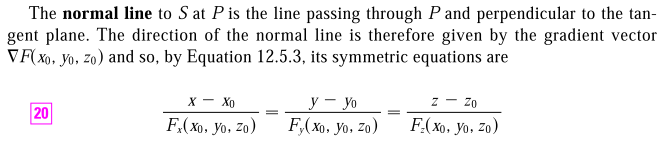## Generalization of the equation for a line

Generalization of the equation for a line

In the 2D world, the line equation is well known:

\begin{align*} \frac{y-y_0}{x-x_0} = k \end{align*}

where k is the slope.

Here is a generalized form:

\begin{align*} \begin{pmatrix} x_1 \\ x_2 \end{pmatrix} - \begin{pmatrix} x_1^* \\ x_2^* \end{pmatrix} &= a \begin{pmatrix} v1 \\ v2 \end{pmatrix} \end{align*}

or more concisely, $x - x^* = av$. This comes in handy when you want to get the equation for the normal line of a level surface, instead of the following awkward presentation,You can use:

\begin{align*} \mathbf{x} - \mathbf{x^*} = a\nabla F \end{align*}